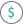#NPV (PQL - xl)

This function calculates the net present value of an investment by using a discount rate and a series of future payments and income

• Library: PQL \ Spreadsheet \ Financial
• Compatibility: Any content (regardless of data source) in the Tabulate spreadsheet module
• Solve: This function can be used with the Solve plug-in: both as part of the objective function and constraint functions

#### Syntax

NPV(rate, value1, OPTIONAL value2)

##### Function Arguments
 Name Description Type Optional rate Rate of discount for one period Number value1 Value for money paid out (as for a payment) are negative numbers; value for money you receive (as for income) are positive numbers Number value2 Value for money paid out (as for a payment) are negative numbers; value for money you receive (as for income) are positive numbers Number Y